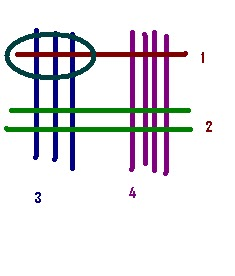How To Multiply Quickly in Aptitude Test

0
11
• 1
•
•
•
•
•
•
•
•
•
1
Share

In this post I am going to tell you, How to Multiply two numbers quickly and accurately.

In several aptitude  test , where calculator is not allowed, this method will surely help you to solve multiplication problems.

Let us say we need to multiply 13X12
So the
Step one is to divide the numbers in the below written format..
The approach is
Step-1
The number 13 is represented in 1 black bar and 3 deep blue bars
and number 12 is represented by 1 deep green bar and two light blue bars.

Step-2

Let us check out the intersection points..

We will start reading from  bottom  right to top left.

Step-3
Counting:
The number of pink circle bottom right is 6
So the result will be XX6
Now look at the middle intersection points…
The green color intersection points are 5
There is no carry so the result will be X56

Now look at the top left intersection marked with red color. That is 1 and we have no carry so the result will be 156
13X12=156

So the common format is

Let us take bit complex example

Let us multiply 12X34

Step 1
12 and 34 are  represented as

Step-2

Let us write it together:

Step-3

Let us start from the bottom left (Intersection count of 4 violet  color and 2 green color) after counting we get 8 so the right most digit will be 8. The result will be XX8Calculation of right number

Step-4

Let us count the mid section. This section is having two parts one bottom 2 green and 3 deep blue intersection the count here is 6 and the upper 1 brown and 4 violet bars which is 4 intersections

So adding up we get 10.Now 10 means 0 will the second  digit will be 0 and 1 will be carry
The result will be (X+1)08Calculation of middle numbers

Step-5 Let us count the top left intersection points
it is only 1 brown bar and 3 deep blue bars. So the total intersection points are 3.Calculating the top left intersection

As we had a carry from step number -4 so the Result will be 3+1=4
The final result will be 408.

Isn’t it easy?? try with bars later you will be able to multiply without drawing these bars. That time it will be on your brain and result will be on your mouth.

Don't miss out!

Receive top technical news, lesson ideas, travel tips and more!

Give it a try. You can unsubscribe at any time.